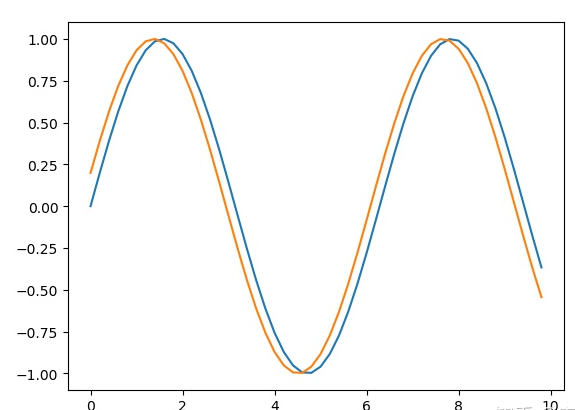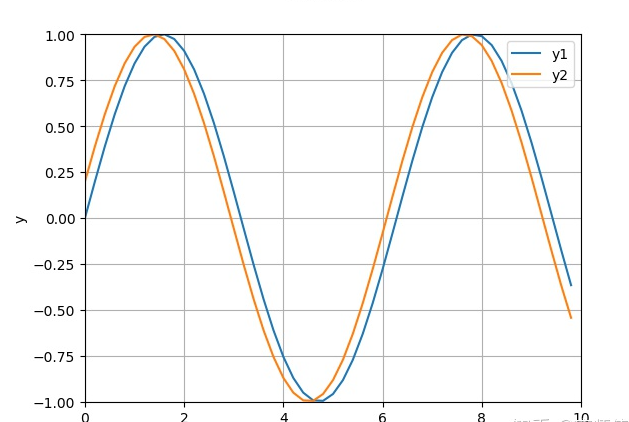# 如何用matlibplot画正弦曲线

```import matplotlib.pyplot as plt
import numpy as np
x = np.arange(0, 10, 0.2)#x的范围0-10 步长0.2
y1 = np.sin(x)#计算y1
y2 = np.sin(x+0.2)#计算y2
fig, ax = plt.subplots()#创建一个figure
ax.plot(x, y1, label='y1')
ax.plot(x, y2, label='y1')
plt.show()``````import matplotlib.pyplot as plt
import numpy as np

x = np.arange(0, 10, 0.2)#x的范围0-10 步长0.2
y1 = np.sin(x)#计算y1
y2 = np.sin(x+0.2)#计算y2
fig, ax = plt.subplots()#创建一个figure
####plot
ax.plot(x, y1, label='y1')
ax.plot(x, y2, label='y2')
####展示图例 legend loc=是用来定义图例的位置的，还有很多选择，大家可以自己尝试
ax.legend(loc = 'upper right')
####定义标题
fig.suptitle('sin curve')
####定义x, y轴的名称
ax.set_xlabel('x')
ax.set_ylabel('y')
####定义x,y轴的显示范围
ax.set_xlim(0, 10)
ax.set_ylim(-1,1)
####打开网格
ax.grid(True)
plt.show()```Python开发开发项目